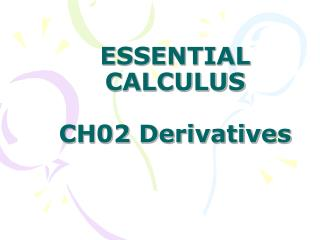DownloadDownload PresentationESSENTIAL CALCULUS CH02 Derivatives

# ESSENTIAL CALCULUS CH02 Derivatives

Download Presentation## ESSENTIAL CALCULUS CH02 Derivatives

- - - - - - - - - - - - - - - - - - - - - - - - - - - E N D - - - - - - - - - - - - - - - - - - - - - - - - - - -
##### Presentation Transcript

1. ESSENTIAL CALCULUSCH02 Derivatives

2. In this Chapter: • 2.1 Derivatives and Rates of Change • 2.2 The Derivative as a Function • 2.3 Basic Differentiation Formulas • 2.4 The Product and Quotient Rules • 2.5 The Chain Rule • 2.6 Implicit Differentiation • 2.7 Related Rates • 2.8 Linear Approximations and Differentials Review

3. Chapter 2, 2.1, P73

4. Chapter 2, 2.1, P73

5. Chapter 2, 2.1, P73

6. Chapter 2, 2.1, P74

7. Chapter 2, 2.1, P74

8. Chapter 2, 2.1, P74

9. Chapter 2, 2.1, P74

10. Chapter 2, 2.1, P74

11. Chapter 2, 2.1, P74

12. Chapter 2, 2.1, P75

13. Chapter 2, 2.1, P75

14. 1 DEFINITION The tangent line to the curve y=f(x) at the point P(a, f(a)) is the line through P with slope m=line Provided that this limit exists. X→ a Chapter 2, 2.1, P75

15. Chapter 2, 2.1, P76

16. Chapter 2, 2.1, P76

17. 4 DEFINITION The derivative of a function f at a number a, denoted by f’(a), is f’(a)=lim if this limit exists. h→ 0 Chapter 2, 2.1, P77

18. f’(a) =lim x→ a Chapter 2, 2.1, P78

19. The tangent line to y=f(X) at (a, f(a)) is the line through (a, f(a)) whose slope is equal to f’(a), the derivative of f at a. Chapter 2, 2.1, P78

20. Chapter 2, 2.1, P78

21. Chapter 2, 2.1, P79

22. Chapter 2, 2.1, P79

23. 6. Instantaneous rate of change=lim ∆X→0 X2→x1 Chapter 2, 2.1, P79

24. The derivative f’(a) is the instantaneous rate of change of y=f(X) with respect to x when x=a. Chapter 2, 2.1, P79

25. 9. The graph shows the position function of a car. Use the shape of the graph to explain your answers to the following questions • What was the initial velocity of the car? • Was the car going faster at B or at C? • Was the car slowing down or speeding up at A, B, and C? • What happened between D and E? Chapter 2, 2.1, P81

26. 10. Shown are graphs of the position functions of two runners, A and B, who run a 100-m race and finish in a tie. (a) Describe and compare how the runners the race. (b) At what time is the distance between the runners the greatest? (c) At what time do they have the same velocity? Chapter 2, 2.1, P81

27. 15. For the function g whose graph is given, arrange the following numbers in increasing order and explain your reasoning. 0 g’(-2) g’(0) g’(2) g’(4) Chapter 2, 2.1, P81

28. the derivative of a function f at a fixed number a: f’(a)=lim h→ 0 Chapter 2, 2.2, P83

29. f’(x)=lim h→ 0 Chapter 2, 2.2, P83

30. Chapter 2, 2.2, P84

31. Chapter 2, 2.2, P84

32. Chapter 2, 2.2, P84

33. 3 DEFINITION A function f is differentiable a if f’(a) exists. It is differentiable on an open interval (a,b) [ or (a,∞) or (-∞ ,a) or (- ∞, ∞)] if it is differentiable at every number in the interval. Chapter 2, 2.2, P87

34. Chapter 2, 2.2, P88

35. Chapter 2, 2.2, P88

36. 4 THEOREM If f is differentiable at a, then f is continuous at a . Chapter 2, 2.2, P88

37. Chapter 2, 2.2, P89

38. Chapter 2, 2.2, P89

39. Chapter 2, 2.2, P89

40. Chapter 2, 2.2, P89

41. (a) f’(-3) (b) f’(-2) (c) f’(-1) • (d) f’(0) (e) f’(1) (f) f’(2) • (g) f’(3) Chapter 2, 2.2, P91

42. 2. (a) f’(0) (b) f’(1) (c) f’’(2) (d) f’(3) (e) f’(4) (f) f’(5) Chapter 2, 2.2, P91

43. Chapter 2, 2.2, P92

44. Chapter 2, 2.2, P92

45. Chapter 2, 2.2, P93

46. Chapter 2, 2.2, P93

47. 33. The figure shows the graphs of f, f’, and f”. Identify each curve, and explain your choices. Chapter 2, 2.2, P93

48. 34. The figure shows graphs of f, f’, f”, and f”’. Identify each curve, and explain your choices. Chapter 2, 2.2, P93

49. Chapter 2, 2.2, P93

50. Chapter 2, 2.2, P93